# Algebra 1 : How to find mode

## Example Questions

### Example Question #61 : How To Find Mode

What is the mode of the following number set?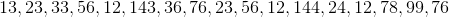Explanation:

What is the mode of the following number set?The mode refers to the most-repeated number in a set. In this case, carefully examine the list and see which number shows up the most.is the mode because it shows uptimes.andonly show up twice, and the rest of the numbers only makeappearance.

### Example Question #61 : How To Find Mode

Refer to the following set of numbers: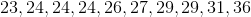Find the mode of the set.Explanation:

The mode of the set is the most repeated number. In this caseis the mode because it is in the set 3 times.

### Example Question #62 : How To Find Mode

Refer to the following set of numbers: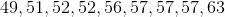Find the mode of the set.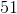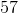Explanation:

The mode of the set is the most repeated number. In this caseis the mode because it is in the set 3 times.

### Example Question #64 : How To Find Mode

Refer to the following set of numbers: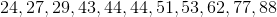Find the mode of the set.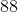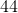Explanation:

The mode of the set is the most repeated number. In this caseis the mode because it is in the set twice.

### Example Question #61 : How To Find Mode

Find the mode of the following set of numbers: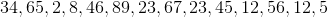.andandandExplanation:

The mode of any set of numbers is the number that occurs the most often in set. In order to find the mode, it's easiest to start by arranging the numbers in the set from least to greatest. The set of numbers we were given isIn order from least to greatest, this set looks like: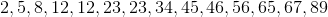Now that our numbers are in order, it's easier for us to see if there are any numbers that show up more than once. In this case, bothandappear in the set two times, and every other number in the set appears only one time. Sinceandappear an equal number of times in the set as the other does, they are both considered to be modes of the set.

So, there are two modes for our set:, and.

### Example Question #65 : How To Find Mode

Find the mode of the following set of numbers: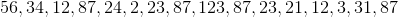.and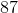andandExplanation:

The mode of any set of numbers is the number in the set that appears most often. In order to find the mode, it is easier to put the numbers in the set in order from least to greatest. The set we were given is.

In order from least to greatest, the set is: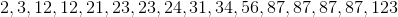Next, we will look through our sequenced set to see if any numbers appear more than one time. In this case, there are several numbers that appear more than once:,, andall appear more than once in our set.

Since we have multiple numbers that appear more than once, now we will look to see how many times each of these numbers appears in the set.- appears two times- appears two times- appears four times

Sinceappears more times in the set than any other number,is the mode of our set.

### Example Question #61 : How To Find Mode

What is the mode of the following set of numbers?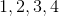No Mode

No Mode

Explanation:

We define mode as the number that appears most often in a set of numbers. Examining the set above, we see that no number appears more times than any other number, and so the set has no mode.

### Example Question #63 : How To Find Mode

What is the mode of the following set of numbers?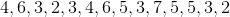Explanation:

We define mode as the number that appears most often in a set of numbers. Examining the set above, we see thatappears the most times and is therefore the mode of the set.

### Example Question #68 : How To Find Mode

What is the mode of the following set of numbers?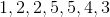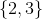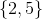No ModeExplanation:

We define mode as the number that appears most often in a set of numbers. Examining the set above, we see thatandappear the most times equally and are therefore the modes of the set.

### Example Question #62 : How To Find Mode

What is the mode of the following set of numbers?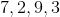No Mode